Courses

# Reactions Involving Liquids and Solids Civil Engineering (CE) Notes | EduRev

## Civil Engineering (CE) : Reactions Involving Liquids and Solids Civil Engineering (CE) Notes | EduRev

The document Reactions Involving Liquids and Solids Civil Engineering (CE) Notes | EduRev is a part of the Civil Engineering (CE) Course Thermodynamics for Engg..
All you need of Civil Engineering (CE) at this link: Civil Engineering (CE)

Reactions involving Liquids and Solids

In many instances of industrially important reactions, the reactants are not only gaseous but are also liquids and / or solids. Such reactions are usually heterogeneous in nature as reactants may exist in separate phases. Some examples include:

• Removal of CO2
• Removal of H from synthesis gas by aqueous solution of potassium carbonate 2
• Air oxidation of aldehydes to acids S by ethanolamine or sodium hydroxide
• Oxidation of cyclohexane to adipic acid • Chlorination of benzene
• Decomposition of CaCO3 to CaO and CO2

In all such instances some species need to dissolve and then diffuse into another phase during the process of reaction. Such reactions therefore require not only reaction equilibria considerations, but that of phase equilibria as well. For simplicity, however we consider here only reaction equilibria of instances where liquid or solid reactive species are involved. The thermodynamic treatment presented below may easily be extended to describe any heterogeneous reaction. The basic relation for the equilibrium constant remains the starting point. By eqn. 8.50 we have: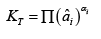..(8.50)

On expanding (by eqn. 6.171):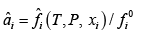..(8.79)

As already mentioned in section 8.1 above, for solids and liquids the usual standard state is the pure solid or liquid at 1 bar [or 1(atm)] and at the temperature (T) of the system. However, unlike in the case of gaseous species, the value of fi o for such a state cannot be 1 bar (or 1 atm), and eqn.(8.50) cannot be reduced to the form simple form of eqn. 8.57.

Liquid-phase reactants On rewriting eqn. 8.79:

On rewriting eqn. 8.79: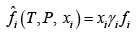Thus: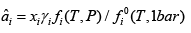..(8.80)

By eqn. 6.115: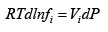Thus on integrating: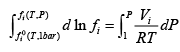..(8.81)

As we have already seen in section 6.10, the liquid phase properties, such as molar volume, are weakly dependent on pressure; hence their variation with respect to pressure may be, for most practical situations, considered negligible. Thus, if one considers that in the last equation the molar volume V i is constant over the range 1 – P bar, one obtains: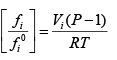..(8.82)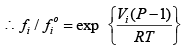..(8.83)

Thus, using eqn. 8.53 in 8.50: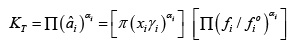..(8.84)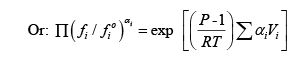Thus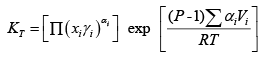..(8.85)

Except for very high pressure the exponential term on the right side of the above equation: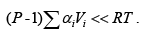Thus one may approximate: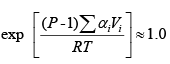Whence: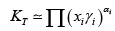...(8.86)

To use the above equation for prediction of the equilibrium composition one needs to employ a suitable activity coefficient model. The activity coefficient models described in section 6.18 are based on physical interactions between molecules. Hence, their use is not expected to be adequately representative of a situation where molecules are subject to specific chemical forces and are chemically transformed due to formation of intermediate reactive complexes. While it is possible to write models for physical interactions it is generally not feasible to establish quantitative relations that describe the microscopic-level interactions between reactive molecular species. Discussions on approaches to solve such problems may be found elsewhere (J.M. Prausnitz, R.N. Lichtenthaler and E.G. Azevedo, Molecular Thermodynamics of Fluid-Phase Equilibria, 3rd ed., Prentice Hall, 1998). Nevertheless, for the purpose of illustrating an approximate solution, one may simplify eqn. 8.86 by assuming ideal solution behavior, wherein γi = 1.0. Hence: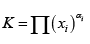...(8.87)

However, since reactive solutions can never be ideal, one way to overcome the difficulty is by defining a reaction equilibrium constant based on molar concentration (say in moles/m3 )  rather than in terms of mole fractions. Thus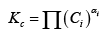...(8.88)

Where, Ci = molar concentration of each species.
It is generally difficult to predict the equilibrium constant Kc, and one needs to use experimentally determine values of such constants in order to predict equilibrium compositions

Solid-phase reactants

Consider a solid reactive species now, for which one again starts from eqn. 8.80: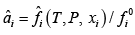...(8.80)

Thus as for a liquid reactant one has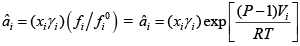As it is for liquid species, Vi  for solids is also small and remains practically constant with pressure,
thus: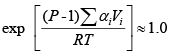In addition, the solid species is typically ‘pure’ as any dissolved gas or liquid (for a multi-phase reaction) is negligible in amount.

Thus xi~ 1.0,→γi= 1.

Therefore, for solids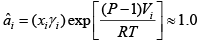...(8.89)

Offer running on EduRev: Apply code STAYHOME200 to get INR 200 off on our premium plan EduRev Infinity!

,

,

,

,

,

,

,

,

,

,

,

,

,

,

,

,

,

,

,

,

,

;